Search by Topic

Filter by: Content type:
Age range:
Challenge level:

There are 27 results

Broad Topics > Transformations and constructions > Constructions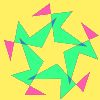Attractive Rotations

Age 11 to 14 Challenge Level:

Here is a chance to create some attractive images by rotating shapes through multiples of 90 degrees, or 30 degrees, or 72 degrees or...Curvy Areas

Age 14 to 16 Challenge Level:

Have a go at creating these images based on circles. What do you notice about the areas of the different sections?Squirty

Age 14 to 16 Challenge Level:

Using a ruler, pencil and compasses only, it is possible to construct a square inside any triangle so that all four vertices touch the sides of the triangle.Triangle Midpoints

Age 14 to 16 Challenge Level:

You are only given the three midpoints of the sides of a triangle. How can you construct the original triangle?Cool as Ice

Age 11 to 16 Challenge Level:

Design and construct a prototype intercooler which will satisfy agreed quality control constraints.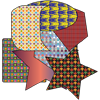Age 7 to 14 Challenge Level:

What shape and size of drinks mat is best for flipping and catching?Constructing Triangles

Age 11 to 14 Challenge Level:

Generate three random numbers to determine the side lengths of a triangle. What triangles can you draw?A Rational Search

Age 14 to 18 Challenge Level:

Investigate constructible images which contain rational areas.Moving Squares

Age 14 to 16 Challenge Level:

How can you represent the curvature of a cylinder on a flat piece of paper?Close to Triangular

Age 14 to 16 Challenge Level:

Drawing a triangle is not always as easy as you might think!Folding Fractions

Age 14 to 16 Challenge Level:

What fractions can you divide the diagonal of a square into by simple folding?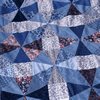Mathematical Patchwork

Age 7 to 14

Jenny Murray describes the mathematical processes behind making patchwork in this article for students.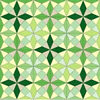A Patchwork Piece

Age 7 to 11 Challenge Level:

Follow the diagrams to make this patchwork piece, based on an octagon in a square.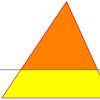Half a Triangle

Age 14 to 16 Challenge Level:

Construct a line parallel to one side of a triangle so that the triangle is divided into two equal areas.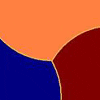Three Tears

Age 14 to 16 Challenge Level:

Construct this design using only compasses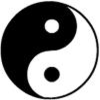Yin Yang

Age 7 to 11 Challenge Level:

Can you reproduce the Yin Yang symbol using a pair of compasses?Pentabuild

Age 16 to 18 Challenge Level:

Explain how to construct a regular pentagon accurately using a straight edge and compass.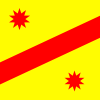Two Points Plus One Line

Age 14 to 16 Challenge Level:

Draw a line (considered endless in both directions), put a point somewhere on each side of the line. Label these points A and B. Use a geometric construction to locate a point, P, on the line,. . . .Golden Construction

Age 16 to 18 Challenge Level:

Draw a square and an arc of a circle and construct the Golden rectangle. Find the value of the Golden Ratio.Circle Scaling

Age 14 to 16 Challenge Level:

Describe how to construct three circles which have areas in the ratio 1:2:3.LOGO Challenge 8 - Rhombi

Age 7 to 16 Challenge Level:

Explore patterns based on a rhombus. How can you enlarge the pattern - or explode it?LOGO Challenge 2 - Diamonds Are Forever

Age 7 to 16 Challenge Level:

The challenge is to produce elegant solutions. Elegance here implies simplicity. The focus is on rhombi, in particular those formed by jointing two equilateral triangles along an edge.Folding Squares

Age 14 to 16 Challenge Level:

The diagonal of a square intersects the line joining one of the unused corners to the midpoint of the opposite side. What do you notice about the line segments produced?The Medieval Octagon

Age 14 to 16 Challenge Level:

Medieval stonemasons used a method to construct octagons using ruler and compasses... Is the octagon regular? Proof please.Pareq Exists

Age 14 to 16 Challenge Level:

Prove that, given any three parallel lines, an equilateral triangle always exists with one vertex on each of the three lines.Gold Again

Age 16 to 18 Challenge Level:

Without using a calculator, computer or tables find the exact values of cos36cos72 and also cos36 - cos72.Kissing

Age 16 to 18 Challenge Level:

Two perpendicular lines are tangential to two identical circles that touch. What is the largest circle that can be placed in between the two lines and the two circles and how would you construct it?